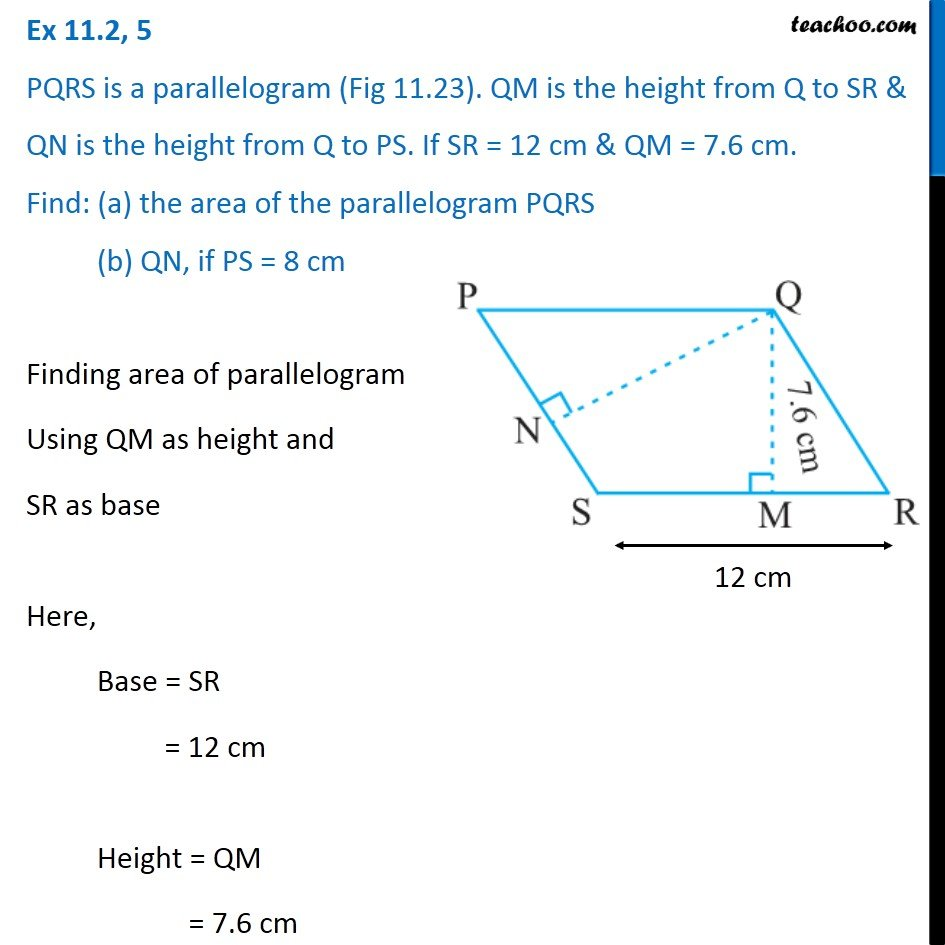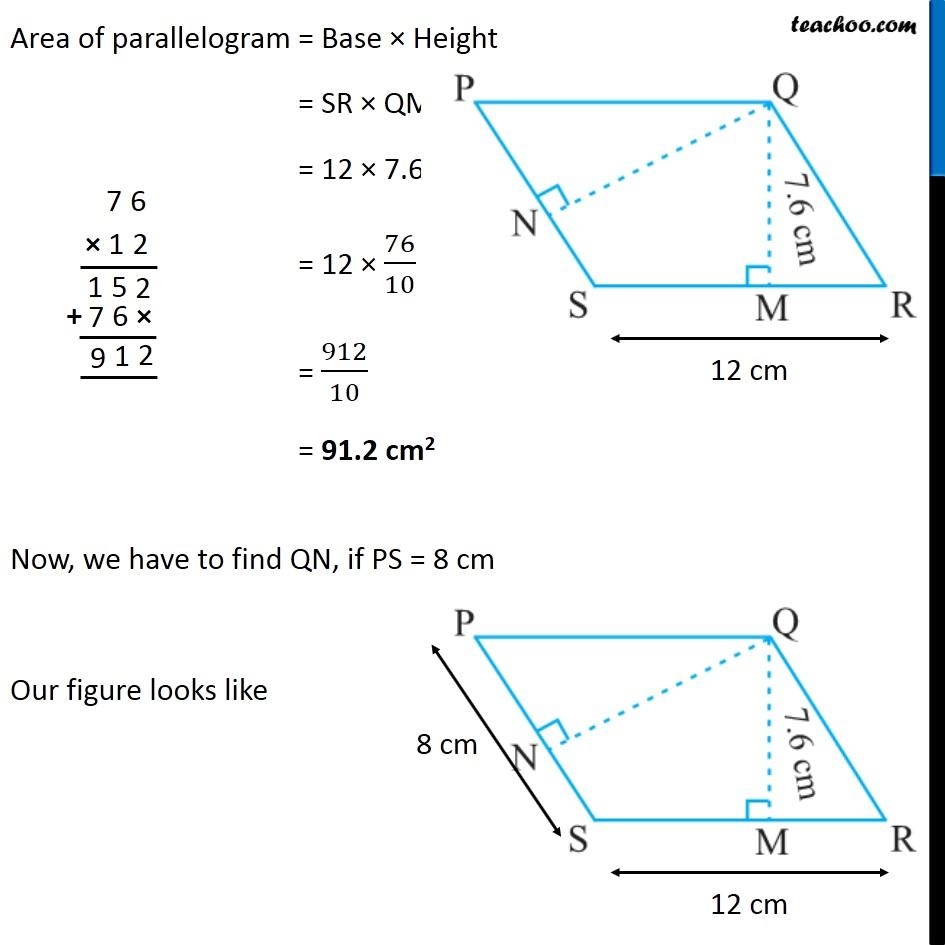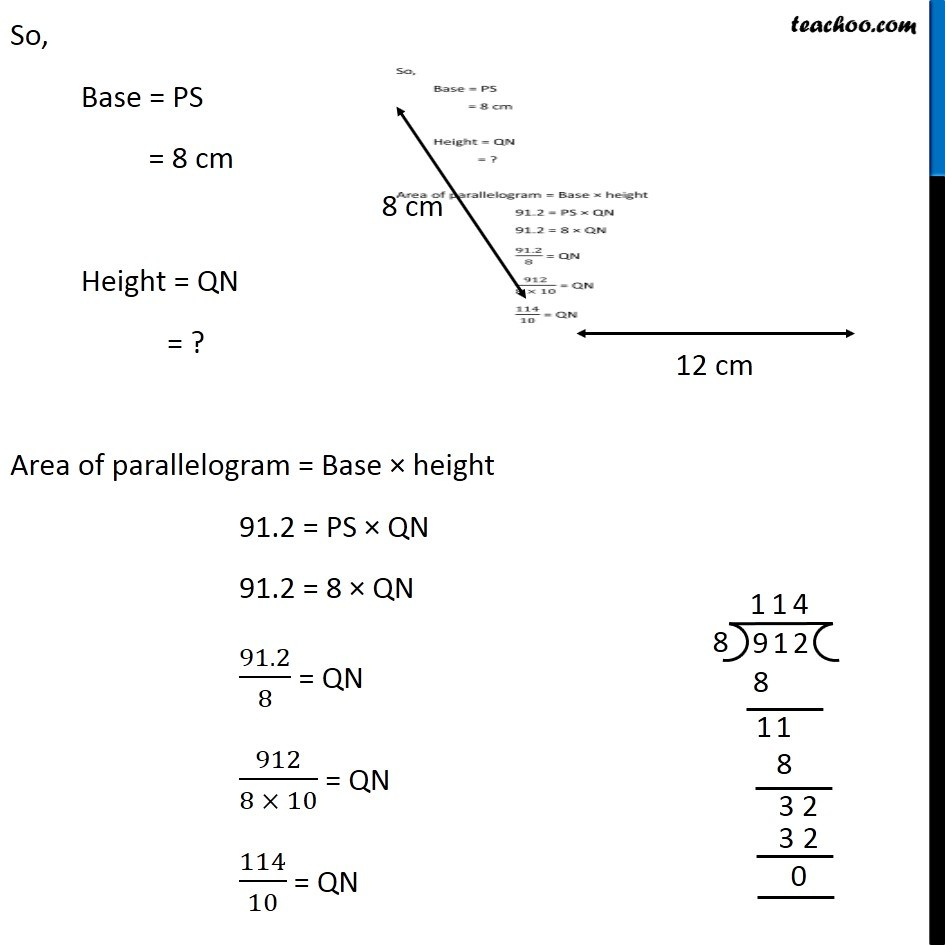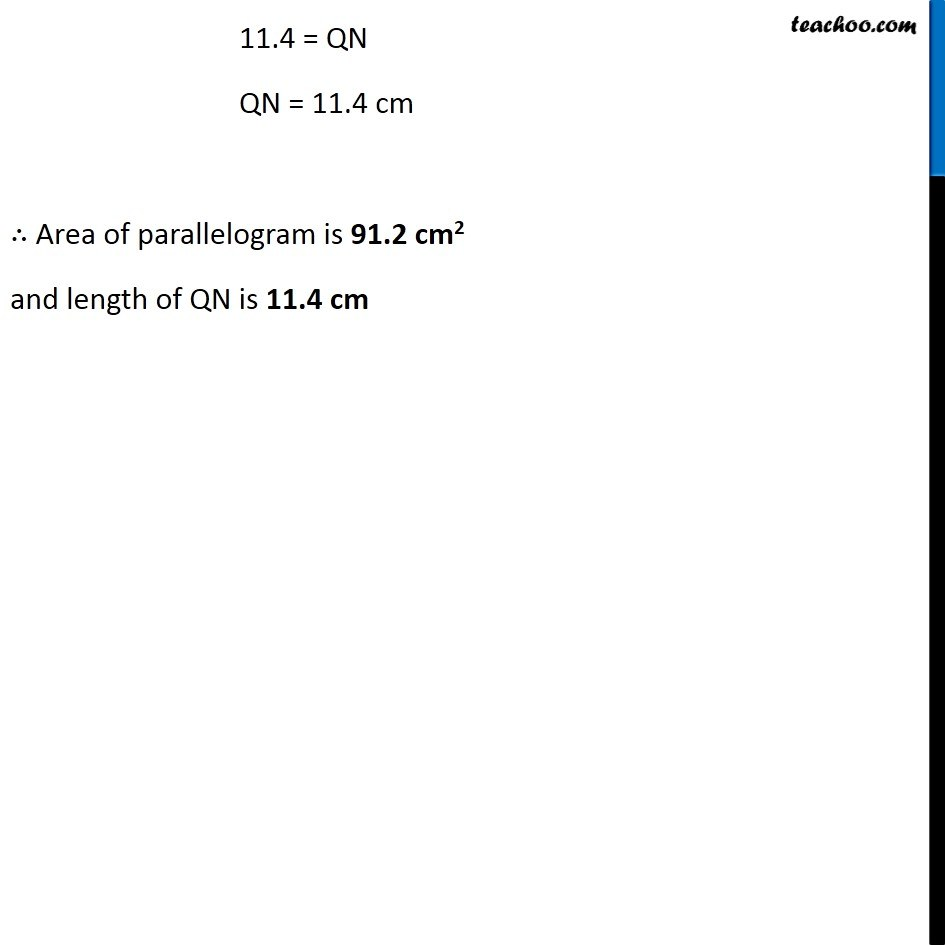Ex 11.2

Chapter 11 Class 7 Perimeter and Area
Serial order wiseGet live Maths 1-on-1 Classs - Class 6 to 12

### Transcript

Ex 11.2, 5 PQRS is a parallelogram (Fig 11.23). QM is the height from Q to SR & QN is the height from Q to PS. If SR = 12 cm & QM = 7.6 cm. Find: (a) the area of the parallelogram PQRS (b) QN, if PS = 8 cm Finding area of parallelogram Using QM as height and SR as base Here, Base = SR = 12 cm Height = QM = 7.6 cm Area of parallelogram = Base × Height = SR × QM = 12 × 7.6 = 12 × 76/10 = 912/10 = 91.2 cm2 Now, we have to find QN, if PS = 8 cm Our figure looks like So, Base = PS = 8 cm Height = QN = ? Area of parallelogram = Base × height 91.2 = PS × QN 91.2 = 8 × QN 91.2/8 = QN 912/(8 × 10) = QN 114/10 = QN 11.4 = QN QN = 11.4 cm ∴ Area of parallelogram is 91.2 cm2 and length of QN is 11.4 cm